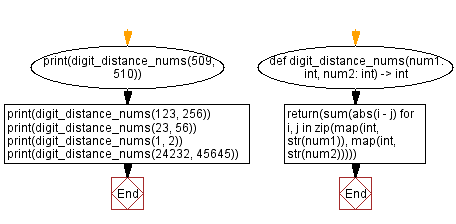﻿ Python: Compute the digit distance between two integers - w3resource# Python: Compute the digit distance between two integers

## Python Basic - 1: Exercise-112 with Solution

Write a Python program to compute the digit distance between two integers.
The digit distance between two numbers is the absolute value of the difference of those numbers.
For example, the distance between 3 and −3 on the number line given by the |3 – (−3) | = |3 + 3 | = 6 units
Digit distance of 123 and 256 is
Since |1 - 2| + |2 - 5| + |3 - 6| = 1 + 3 + 3 = 7

Sample Solution:

Python Code:

``````def digit_distance_nums(n1, n2):
return sum(map(int,str(abs(n1-n2))))
print(digit_distance_nums(123, 256))
print(digit_distance_nums(23, 56))
print(digit_distance_nums(1, 2))
print(digit_distance_nums(24232, 45645))
``````

Sample Output:

```7
6
1
11
```

Flowchart:Python Code Editor:

Have another way to solve this solution? Contribute your code (and comments) through Disqus.

What is the difficulty level of this exercise?

Test your Programming skills with w3resource's quiz.

﻿

## Python: Tips of the Day

What is the difference between Python's list methods append and extend?

append: Appends object at the end.

```x = [1, 2, 3]
x.append([4, 5])
print (x)
```

Output:

```[1, 2, 3, [4, 5]]
```

extend: Extends list by appending elements from the iterable.

```x = [1, 2, 3]
x.extend([4, 5])
print (x)
```

Output:

```[1, 2, 3, 4, 5]
```

Ref: https://bit.ly/2AZ6ZFq